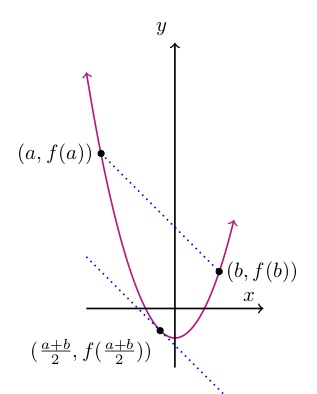# Mean Value Theorem

The mean value theorem says that if you look at a continuous, differentiable function on some limited interval, there must be at least one point in that interval at which the slope of the tangent line to that function equals the slope of the line connecting the points on the function at the ends of the interval. Stated more formally, for a function that is continuous on the closed interval and differentiable on the open interval the slope of the secant line between and equals the derivative of the function at at least one point  within the interval.

## Question

Function is a second degree function:Find point on interval  such that  equals the slope of the secant line from  to .

## Solution

The mean value theorem is all about the secant line’s slope and the function’s derivative. See what you can say about both.

## Secant line’s slope

slope = (change in y)/(change in x) =

Secant slope=

## Function’s derivative

Is there a value of x for which these equal? Call that value and try to find it.

This is interesting. It means that for a second-degree function — a parabola — the slope of the secant line between two points equals the instantaneous slope (that is, the slope of the tangent line) of the function halfway through the interval.f(b); tangent and secant lines slope downward” width=”220″ height=”274″ />Get tutored online.

Jill tutors

algebra, geometry, and calculus

online.